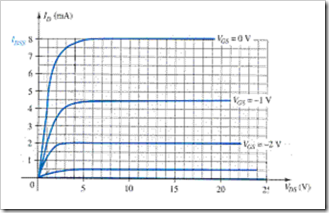# Practice Quiz in Field Effect Transistor Devices Part 3

(Last Updated On: February 3, 2020)

This is the Online Practice Quiz in Field Effect Transistor Devices Part 3 from the book, Electronic Devices and Circuit Theory 10th Edition by Robert L. Boylestad. If you are looking for a reviewer in Electronics Engineering this will definitely help. I can assure you that this will be a great help in reviewing the book in preparation for your Board Exam. Make sure to familiarize each and every questions to increase the chance of passing the ECE Board Exam.

### Continue Part III of the Online Practice Quiz

#### Quiz in Field Effect Transistor Devices

Question 21. A BJT is a _____-controlled device. The JFET is a _____ - controlled device.

A. voltage, voltage

B. voltage, current

C. current, voltage

D. current, current

Question 22. Refer to the following characteristic curve. Calculate the resistance of the FET at VGS = –0.25 V if ro = 10 kΩ.A. 1.1378 kΩ

B. 113.78 Ω

C. 11.378 Ω

D. 11.378 kΩ

Question 23. Which of the following is (are) the terminal(s) of a field-effect transistor (FET).

A. Drain

B. Gate

C. Source

D. All of these

Question 24. Which of the following represent(s) the cutoff region for an FET?

A. ID = 0 mA

B. VGS = VP

C. IG = 0

D. All of these

Question 25. Which of the following FETs has the lowest input impedance?

A. JFET

B. MOSFET depletion-type

C. MOSFET enhancement-type

D. None of these

Question 26. At which of the following is the level of VDS equal to the pinch-off voltage?

A. When ID becomes equal to IDSS

B. When VGS is zero volts

C. IG is zero

D. All of these

Question 27. Which of the following applies to MOSFETs?

A. No direct electrical connection between the gate terminal and the channel

B. Desirable high input impedance

C. Uses metal for the gate, drain, and source connections

D. All of these

Question 28. What is the range of an FET's input impedance?

A. 10 Ω to 1 kΩ

B. 1 kΩ to 10 kΩ

C. 50 kΩ to 100 kΩ

D. 1 M Ω to several hundred MΩ

Question 29. What is the level of drain current ID for gate-to-source voltages VGS less than (more negative than) the pinch-off level?

A. zero amperes

B. IDSS

C. Negative value

D. Undefined

Question 30. At which of the following condition(s) is the depletion region uniform?

A. No bias

B. VDS > 0 V

C. VDS = VP

D. None of these

### More Practice Quiz in Field Effect Transistor Devices

Practice Quiz Part 1

Practice Quiz Part 2

Practice Quiz Part 3

Practice Quiz Part 4

Practice Quiz Part 5

Practice Quiz Part 6

### See: Complete List of Practice Quizzes

Note: After taking this particular quiz, you can proceed to check all the topics.

Please do Subscribe on YouTube!

P inoyBIX educates thousands of reviewers and students a day in preparation for their board examinations. Also provides professionals with materials for their lectures and practice exams. Help me go forward with the same spirit.

“Will you subscribe today via YOUTUBE?”

Subscribe